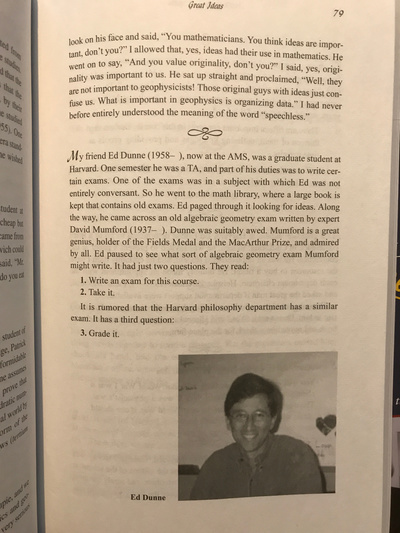# Is there a fair Peer Exam?

With final exams coming up, we should muse a bit about the format. Wouldn't it be nice if the class could write and grade a good fair exam by itself and the teacher does not have to grade anything?

As mentioned in Unit 12, there is an entry in the Apocrypha of Steven Krantz (there are two volumes, it is in the first volume issued 2002). According to this entry David Mumford (an algebraic geometry legend) has made the following problem Problem 1: Write an exam. Problem 2: Take the exam. An adaptation done by the philosophy department then added Problem 3: Grade the exam. Obviously this ``Harvard method" does not work: everybody concerned with having decent grades would just make a very easy problem. Is there a peer solution which produces a good exam which challenges everybody but which is also fair? Lets try to formulate the problem more clearly:

 Assume n=55 students have to take an exam. The exam consists of 10 problems with 10 points each. Every student will have to submit an exam with 10 problems, every student should have 10 problems to solve and m=10 problems to grade. We want the exam to be fair and balanced with an 80 percent average score. A student's score can depend on a formula which depend on the score table as well as the grading table of each student. The formula is known to everybody ahead of time. No additional staff nor other criteria should be involved. How can we make this fair ``peer only" exam?

Here is a suggestion:
As required, every student submits 10 problems. The problems are shuffled and randomly assigned to the class so that each student gets a random set of 10 problems (we just make sure that nobody has to solve his or her own problem). Also the grading assignment is randomly assigned again so that no collision appears. The grade of student x is now the graded exam grade minus the M(x), which is the average deviation from 80 among all problems submitted by student x.

So, if a student submits an easier exam which reaches an average score of 90, and has himself an exam score of 95, then actual score is 95-10 = 85. If a student submits a hard exam reaching an average score of 75 and scores herself 92, her grade is 93-5=88.

The method actually tests more than knowing the material only. It also achieves more. In order to do well, a student not only has to understand the material but also has to have an idea how well the entire class understands the material.The books of Krantz (with lemon tree)And a page from the first book.